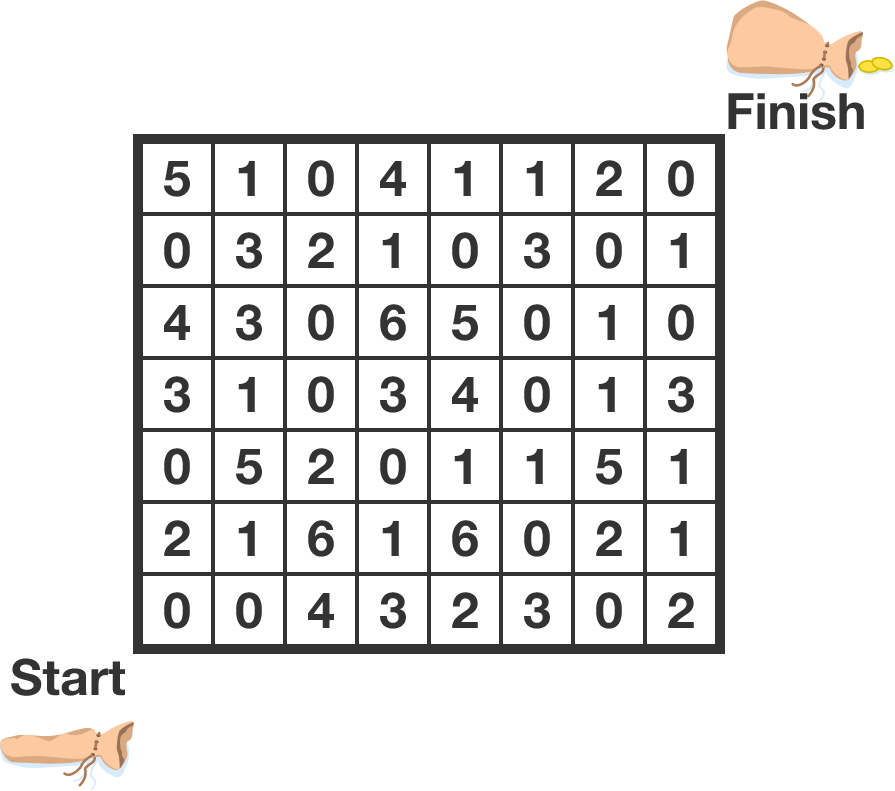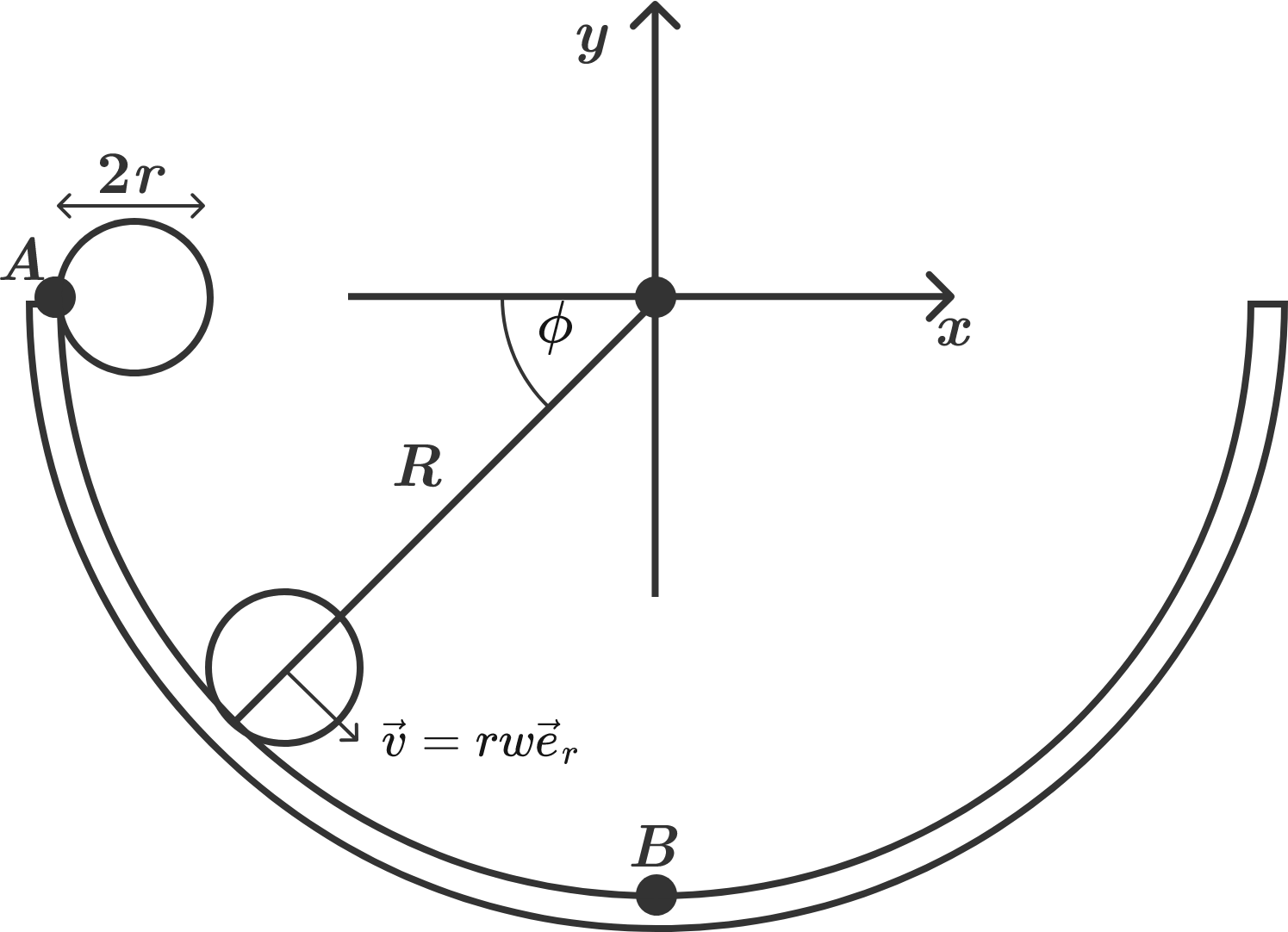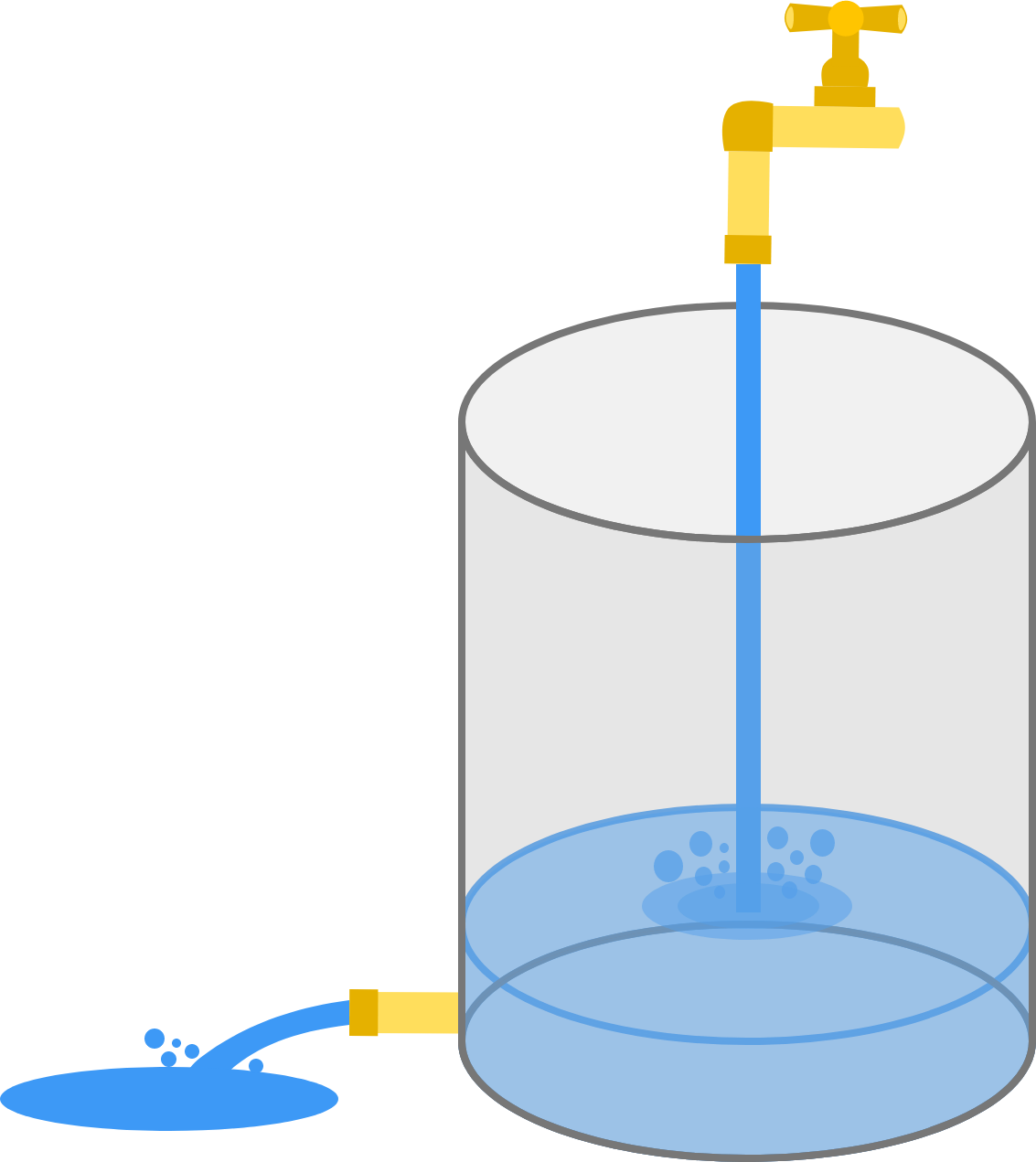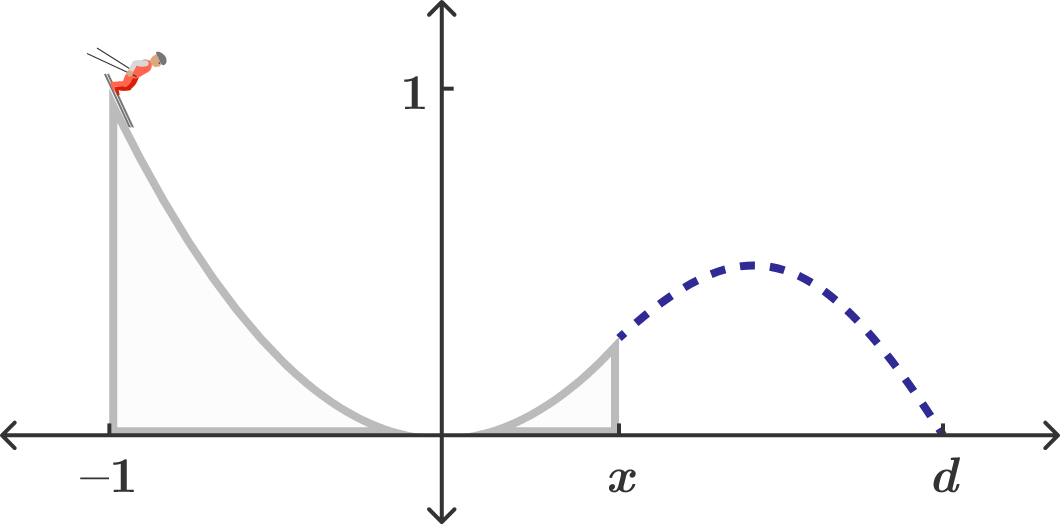# Problems of the Week

Contribute a problem

# 2017-09-25 IntermediateIn the grid above, the number of gold coins in each cell is given. Adam needs to start in the bottom-left square and move to the top-right square, moving only up and to the right.

What is the maximum number of gold coins he can collect?

This can be solved using a recursive approach (dynamic programming).

• I've set up the grid (coins), where the bottom-left cell is (0,0) and the top-right is (7,6); i.e., coins[(1,2)] = 5.
• I've also computed max_coins, the maximum number of coins Adam can collect along the way to a given cell, for the first column and row.
• You need to fill in the logic in line 19 - the recursive step for max_coins for the rest of the cells - and run the code!

Hint: What are the possible states just before you reach a given cell?

from brilliant import goldgrid
coins = goldgrid.grid()
height, width = goldgrid.height(coins), goldgrid.width(coins)

#Initializing the dictionary for the maximum coins up to a certain cell
max_coins = {(0,0) : coins[(0,0)]}

#Computing the max_coins values for the first column and row
#The maximum coins is the maximum for the cell prior to it, plus the coins in that cell
for row in range(1,height):
max_coins[(0,row)] = max_coins[(0,row-1)] + coins[(0,row)]

for column in range(1,width):
max_coins[(column,0)] = max_coins[(column-1,0)] + coins[(column,0)]

#Using recursion / dynamic programming to find the remaining maximums
for row in range(1,height):
for column in range(1,width):
max_coins[(column,row)] = #FILL ME IN

#Print the maximum value for the top right cell
print(max_coins[(width-1,height-1)])
Python 3
You need to be connected to run code

Bonuses: How can you initialize max_coins differently to avoid the separate cases for the first column and row, and just have a single recursive loop? Also, can you modify the code to return the actual path that gives the maximum gold?

$\left \lfloor \dfrac{1^2}{2017} \right \rfloor , \, \left \lfloor \dfrac{2^2}{2017} \right \rfloor , \, \left \lfloor \dfrac{3^2}{2017} \right \rfloor , \, \ldots , \, \left \lfloor \dfrac{2017^2}{2017} \right \rfloor$

How many distinct numbers are there in the list of numbers above?


Notation: $\lfloor \cdot \rfloor$ denotes the floor function.

Consider an immovable bowl in the shape of a perfect hemisphere with radius $R = 19 \text{ cm},$ as shown in the diagram below. Now, place a spherical marble with radius $r = 1 \text{ cm}$ on the edge of the bowl $($point $A)$ and let it roll.

How long does the marble take to reach the bottom of the bowl $($point $B)?$Assume that $g = \SI[per-mode=symbol]{10}{\meter\per\second\squared}.$You fill a cylindrical water tank, with the bottom securely closed, with 5 minutes of constant water flow from the top.

You turn off the flow at the top, and let the full tank drain freely by the force of gravity, through a pipe on the bottom. It takes 10 minutes to empty the tank.

If this pipe is accidentally left open when the tank is being filled, how long will it take to fill the tank?You're building a ski jump for the upcoming Winter Olympics and it's been decided that the hill and ramp will be formed from the shape of a parabola, so that at any $x,$ the height is given by $h(x) = x^2.$

If the skiers are to start from $x = -1,$ where should the end of the ramp be placed so as to maximize the distance $d$ from the origin to the skier's landing point?